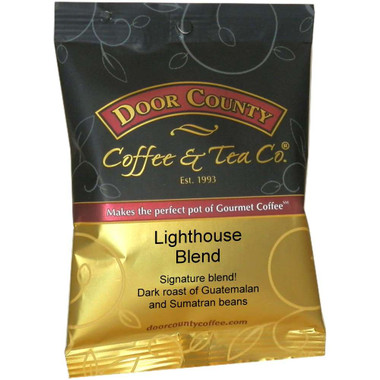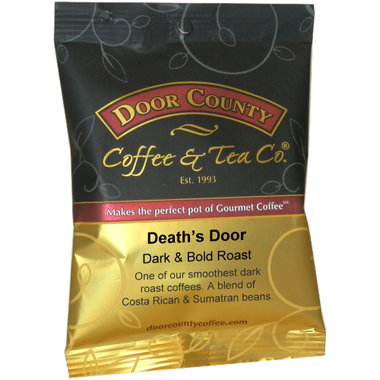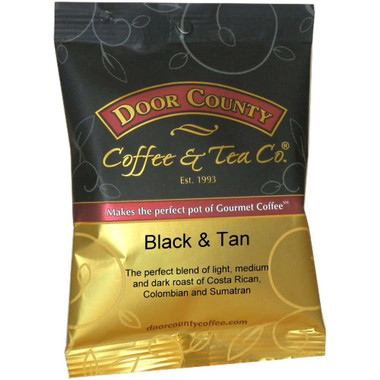•Quantity Decrease Quantity Increase Quantity Decrease Quantity
•Quantity Decrease Quantity Increase Quantity Decrease Quantity
•Quantity Decrease Quantity Increase Quantity Decrease Quantity
•Quantity Decrease Quantity Increase Quantity Decrease Quantity
•Quantity Decrease Quantity Increase Quantity Decrease Quantity
•Quantity Decrease Quantity Increase Quantity Decrease Quantity
•Quantity Decrease Quantity Increase Quantity Decrease Quantity
•Quantity Decrease Quantity Increase Quantity Decrease Quantity
•\$15.99
\$18.99
•Quantity Decrease Quantity Increase Quantity Decrease Quantity
\$49.95
\$54.95
•Quantity Decrease Quantity Increase Quantity Decrease Quantity
\$49.95
\$54.95
•Quantity Decrease Quantity Increase Quantity Decrease Quantity
\$54.95
\$59.95